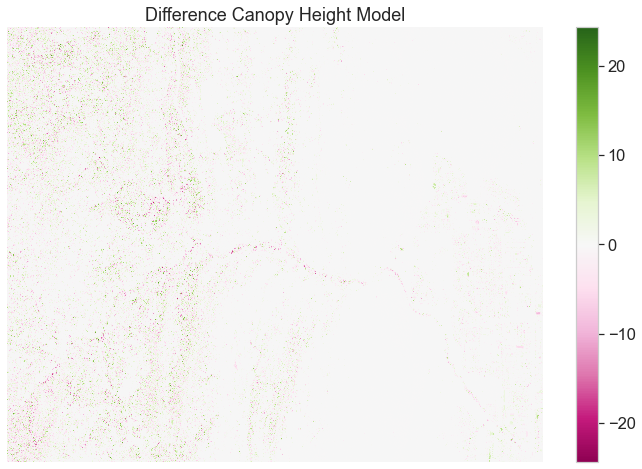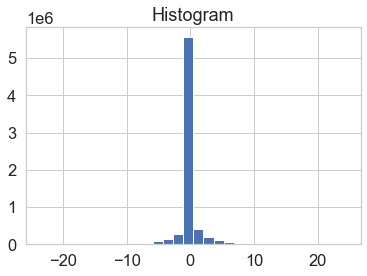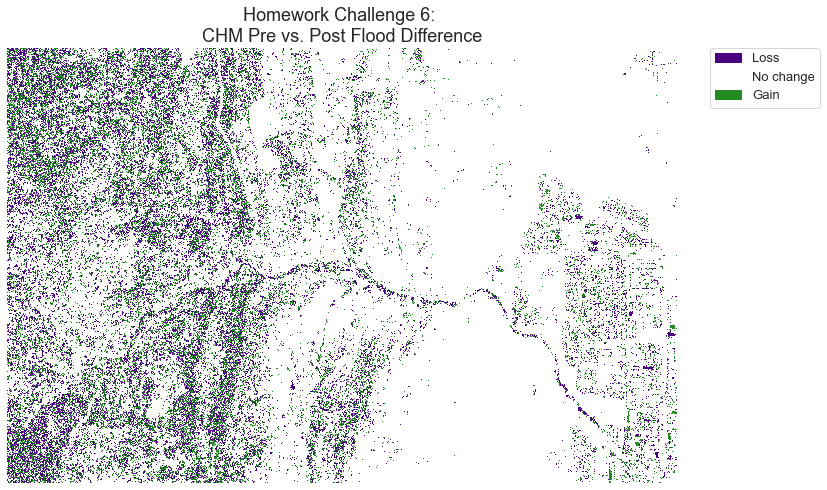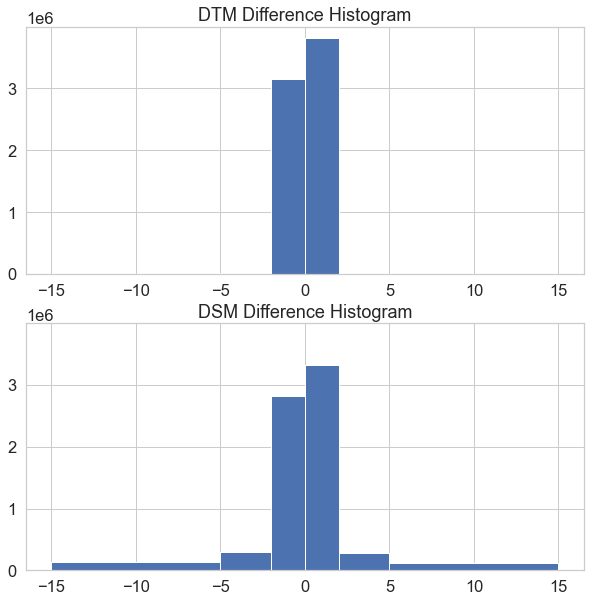# Introduction to Spatial Raster Data in Open Source Python

## Welcome to Week 12!

Welcome to Week 12 of the Earth Analytics Bootcamp course! This week, you will write Python code in Jupyter Notebook to work with spatial raster data.

## Learning Objectives

After completing the lessons for Week 12, you will be able to:

• Open and plot raster data using xarray in Python
• Crop raster data using rioxarray
• Manually classify raster data
• Perform basic raster math

## Earth Data Science Textbook Readings

Please read the following chapters of the Intermediate to Earth Data Science online textbook to support completing this week’s assignment:

Please read and watch the following chapters in the of the Intermediate Earth Data Science online textbook to support completing this week’s assignment:

## Example Homework Plots

Below are example versions of the plots you will create for your homework.Plots of lidar min and max vs insitu min and max with a 1:1 line a regression fit for the NEON SJER field site.Histogram of values from the raster plot of the difference in the canopy height model.Categorized raster of areas that gained or lost elevation after the flood.
# HW plot 6
# PLOT 3: pre/post DTM difference raster histogram

bins = [-15, -5, -2, 0, 2, 5, 15]

diff_dtm = post_dtm_xr_cl - pre_dtm_xr_cl
diff_dsm = post_dsm_xr_cl - pre_dsm_xr_cl

f, (ax1, ax2) = plt.subplots(2, 1, figsize=(10, 10), sharey=True)
diff_dtm.plot.hist(bins=bins,
ax=ax1)
ax1.set(title="DTM Difference Histogram")

diff_dsm.plot.hist(bins=bins,
ax=ax2)
ax2.set(title="DSM Difference Histogram")
plt.show()Two histogram plots. The top plot shows the values found in the difference raster of the digital terrain model. The bottom plot shows the values found in the difference raster of the digital surface model.